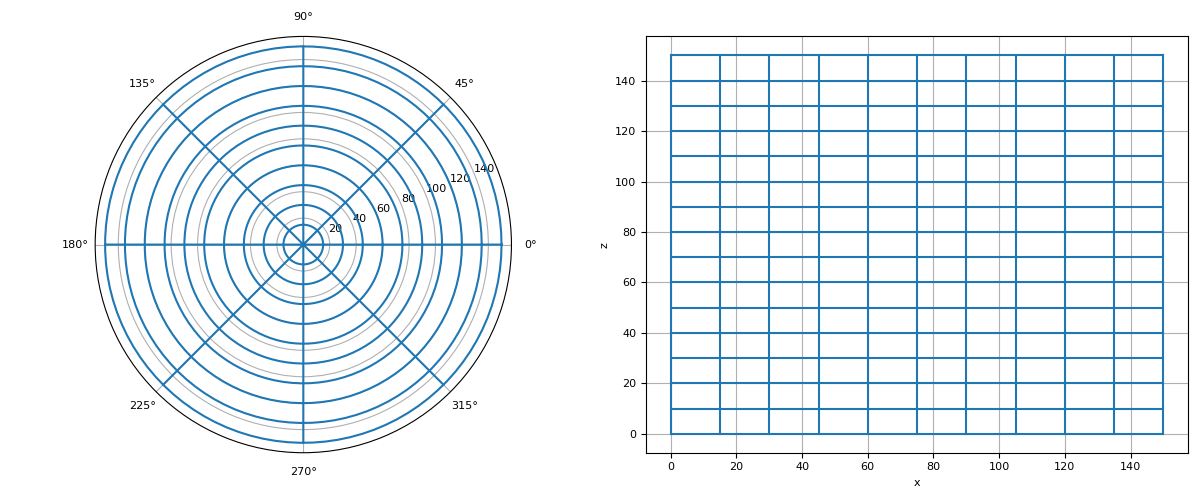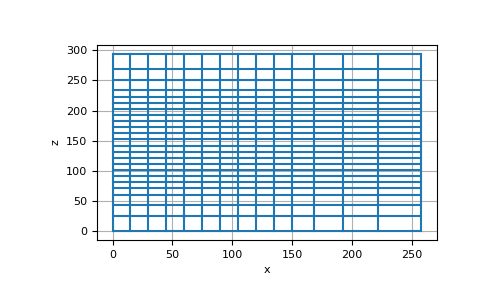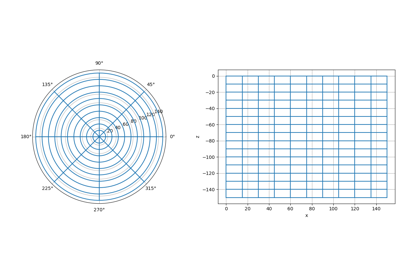# discretize.CylindricalMesh¶class discretize.CylindricalMesh(h, origin=None, cartesian_origin=None, **kwargs)[source]

Class for cylindrical meshes.

CylindricalMesh is a mesh class for problems with rotational symmetry. It supports both cylindrically symmetric and 3D cylindrical meshes where the azimuthal discretization is user-defined. In discretize, the coordinates for cylindrical meshes are as follows:

• x: radial direction ($$r$$)

• y: azimuthal direction ($$\phi$$)

• z: vertical direction ($$z$$)

Parameters
h(dim) iterable of int, numpy.ndarray, or tuple

Defines the cell widths along each axis. The length of the iterable object is equal to the dimension of the mesh (1, 2 or 3). For a 3D mesh, the list would have the form [hr, hphi, hz] . Note that the sum of cell widths in the phi direction must equal $$2\pi$$. You can also use a flat value of hphi = 1 to define a cylindrically symmetric mesh.

Along each axis, the user has 3 choices for defining the cells widths:

• int -> A unit interval is equally discretized into N cells.

• numpy.ndarray -> The widths are explicity given for each cell

• the widths are defined as a list of tuple of the form (dh, nc, [npad]) where dh is the cell width, nc is the number of cells, and npad (optional)is a padding factor denoting exponential increase/decrease in the cell width or each cell; e.g. [(2., 10, -1.3), (2., 50), (2., 10, 1.3)]

origin(dim) iterable, default: 0

Define the origin or ‘anchor point’ of the mesh; i.e. the bottom-left-frontmost corner. By default, the mesh is anchored such that its origin is at [0, 0, 0].

For each dimension (r, theta or z), The user may set the origin 2 ways:

• a scalar which explicitly defines origin along that dimension.

• {‘0’, ‘C’, ‘N’} a str specifying whether the zero coordinate along each axis is the first node location (‘0’), in the center (‘C’) or the last node location (‘N’).

Examples

To create a general 3D cylindrical mesh, we discretize along the radial, azimuthal and vertical axis. For example:

>>> from discretize import CylindricalMesh
>>> import matplotlib.pyplot as plt
>>> import numpy as np

>>> ncr = 10  # number of mesh cells in r
>>> ncp = 8   # number of mesh cells in phi
>>> ncz = 15  # number of mesh cells in z
>>> dr = 15   # cell width r
>>> dz = 10   # cell width z

>>> hr = dr * np.ones(ncr)
>>> hp = (2 * np.pi / ncp) * np.ones(ncp)
>>> hz = dz * np.ones(ncz)
>>> mesh1 = CylindricalMesh([hr, hp, hz])

>>> mesh1.plot_grid()
>>> plt.show()For a cylindrically symmetric mesh, the disretization along the azimuthal direction is set with a flag of 1. This reduces the size of numerical systems given that the derivative along the azimuthal direction is 0. For example:

>>> ncr = 10      # number of mesh cells in r
>>> ncz = 15      # number of mesh cells in z
>>> dr = 15       # cell width r
>>> dz = 10       # cell width z
>>> exp_r = 1.25  # expansion rate of padding cells in r
>>> exp_z = 1.25  # expansion rate of padding cells in z


A value of 1 is used to define the discretization in phi for this case.

>>> hr = [(dr, ncr), (dr, npad_r, exp_r)]
>>> mesh2 = CylindricalMesh([hr, 1, hz])

>>> mesh2.plot_grid()
>>> plt.show()


(png, pdf)Attributes

 area area has been deprecated. areaFx areaFx has been deprecated. areaFy areaFy has been deprecated. areaFz areaFz has been deprecated. average_cell_to_edge Averaging operator from cell centers to edges (scalar quantities). average_cell_to_face Averaging operator from cell centers to faces (scalar quantities). average_cell_vector_to_face Averaging operator from cell centers to faces (vector quantities). average_edge_to_cell Averaging operator from edges to cell centers (scalar quantities). average_edge_to_cell_vector Averaging operator from edges to cell centers (vector quantities). average_edge_to_face_vector Averaging operator from edges to faces (vector quantities). average_edge_x_to_cell Averaging operator from x-edges to cell centers (scalar quantities). average_edge_y_to_cell Averaging operator from y-edges to cell centers (scalar quantities). average_edge_z_to_cell Averaging operator from z-edges to cell centers (scalar quantities). average_face_to_cell Averaging operator from faces to cell centers (scalar quantities). average_face_to_cell_vector Averaging operator from faces to cell centers (vector quantities). average_face_x_to_cell Averaging operator from x-faces to cell centers (scalar quantities). average_face_y_to_cell Averaging operator from y-faces to cell centers (scalar quantities). average_face_z_to_cell Averaging operator from z-faces to cell centers (scalar quantities). average_node_to_cell Averaging operator from nodes to cell centers (scalar quantities). average_node_to_edge Averaging operator from nodes to edges (scalar quantities). average_node_to_face Averaging operator from nodes to faces (scalar quantities). axis_u Deprecated since version 0.7.0. axis_v Deprecated since version 0.7.0. axis_w Deprecated since version 0.7.0. boundary_edge_vector_integral Represents the operation of integrating a vector function on the boundary boundary_edges Boundary edge locations boundary_face_outward_normals Outward normal vectors of boundary faces boundary_face_scalar_integral Represents the operation of integrating a scalar function on the boundary boundary_faces Boundary face locations boundary_node_vector_integral Represents the operation of integrating a vector function dotted with the boundary normal boundary_nodes Boundary node locations cartesianOrigin cartesianOrigin has been deprecated. cartesian_origin Cartesian origin of the mesh cellGrad cellGrad has been deprecated. cellGradBC cellGradBC has been deprecated. cellGradx cellGradx has been deprecated. cellGrady cellGrady has been deprecated. cellGradz cellGradz has been deprecated. cell_centers Return gridded cell center locations cell_centers_x Returns the x-positions of cell centers along the x-direction cell_centers_y Returns the y-positions of cell centers along the y-direction (azimuthal) cell_centers_z Return z-coordinates of the cell centers along the z-direction cell_gradient Cell gradient operator (cell centers to faces) cell_gradient_BC Boundary conditions matrix for the cell gradient operator (Deprecated) cell_gradient_x X-derivative operator (cell centers to x-faces) cell_gradient_y Y-derivative operator (cell centers to y-faces) cell_gradient_z Z-derivative operator (cell centers to z-faces) cell_volumes Volumes of all mesh cells dim The dimension of the mesh (1, 2, or 3). edge edge has been deprecated. edgeCurl edgeCurl has been deprecated. edgeEx edgeEx has been deprecated. edgeEy edgeEy has been deprecated. edgeEz edgeEz has been deprecated. edge_curl Edge curl operator (edges to faces) edge_lengths Lengths of all mesh edges edge_tangents Unit tangent vectors for all mesh edges edge_x_lengths x-edge lengths for entire mesh edge_y_lengths arc-lengths of y-edges for entire mesh edge_z_lengths z-edge lengths for entire mesh edges Gridded edge locations edges_x Gridded x-edge locations edges_y Gridded y-edge (azimuthal edge) locations edges_z Gridded z-edge (vertical edge) locations faceDiv faceDiv has been deprecated. faceDivx faceDivx has been deprecated. faceDivy faceDivy has been deprecated. faceDivz faceDivz has been deprecated. face_areas Face areas for the entire mesh face_divergence Face divergence operator (faces to cell-centres) face_normals Unit normal vectors for all mesh faces face_x_areas x-face areas for the entire mesh face_x_divergence X-derivative operator (x-faces to cell-centres) face_y_areas y-face areas for the entire mesh face_y_divergence Y-derivative operator (y-faces to cell-centres) face_z_areas z-face areas for the entire mesh face_z_divergence Z-derivative operator (z-faces to cell-centres) faces Gridded face locations faces_x Gridded x-face (radial face) locations faces_y Gridded y-face locations faces_z Gridded z-face locations h Cell widths along each axis direction h_gridded Return dimensions of all mesh cells as staggered grid. hx Width of cells in the x direction hy Width of cells in the y direction hz Width of cells in the z direction isSymmetric isSymmetric has been deprecated. is_symmetric Validates whether mesh is symmetric. nCx Number of cells in the x direction nCy Number of cells in the y direction nCz Number of cells in the z direction nNx Number of nodes in the x-direction nNy Number of nodes in the y-direction nNz Number of nodes in the z-direction n_cells Total number of cells in the mesh. n_edges Total number of edges in the mesh n_edges_per_direction The number of edges in each direction n_edges_x Number of x-edges in the mesh n_edges_y Number of y-edges in the mesh n_edges_z Total number of z-edges in the mesh n_faces Total number of faces in the mesh n_faces_per_direction The number of faces in each axis direction n_faces_x Number of x-faces in the mesh n_faces_y Number of y-faces in the mesh n_faces_z Number of z-faces in the mesh n_nodes Returns total number of mesh nodes nodalGrad nodalGrad has been deprecated. nodalLaplacian nodalLaplacian has been deprecated. nodal_gradient Nodal gradient operator (nodes to edges) nodal_laplacian Nodal scalar Laplacian operator (nodes to nodes) nodes Gridded node locations nodes_x Returns the x-positions of nodes along the x-direction (radial) nodes_y Returns the y-positions of nodes along the y-direction (azimuthal) nodes_z Return z-coordinates of the nodes along the z-direction normals normals has been deprecated. orientation Rotation matrix defining mesh axes relative to Cartesian origin Origin or 'anchor point' of the mesh project_edge_to_boundary_edge Projection matrix from all edges to boundary edges. project_face_to_boundary_face Projection matrix from all faces to boundary faces. project_node_to_boundary_node Projection matrix from all nodes to boundary nodes. reference_is_rotated Indicates whether mesh uses standard coordinate axes reference_system Coordinate reference system rotation_matrix Alias for orientation shape_cells The number of cells in each coordinate direction. shape_edges_x Number of x-edges along each axis direction shape_edges_y Number of y-edges along each axis direction shape_edges_z Number of z-edges along each axis direction shape_faces_x Number of x-faces along each axis direction shape_faces_y Number of y-faces along each axis direction shape_faces_z Number of z-faces along each axis direction shape_nodes Returns the number of nodes along each axis stencil_cell_gradient Stencil for cell gradient operator (cell centers to faces) stencil_cell_gradient_x Differencing operator along x-direction (cell centers to x-faces) stencil_cell_gradient_y Differencing operator along y-direction (cell centers to y-faces) stencil_cell_gradient_z Differencing operator along z-direction (cell centers to z-faces) tangents tangents has been deprecated. vectorCCx vectorCCx has been deprecated. vectorCCy vectorCCy has been deprecated. vectorCCz vectorCCz has been deprecated. vectorNx vectorNx has been deprecated. vectorNy vectorNy has been deprecated. vectorNz vectorNz has been deprecated. vol vol has been deprecated. x0 An alias for the origin

Methods

 cartesianGrid(*args, **kwargs) cartesianGrid has been deprecated. cartesian_grid([location_type, theta_shift]) Takes a grid location ('CC', 'N', 'Ex', 'Ey', 'Ez', 'Fx', 'Fy', 'Fz') and returns that grid in cartesian coordinates cell_gradient_weak_form_robin([alpha, beta, ...]) Robin conditions for weak form of the cell gradient operator (cell centers to faces) Make a copy of the current mesh deserialize(items, **kwargs) Create this mesh from a dictionary of attributes edge_divergence_weak_form_robin([alpha, ...]) Robin conditions for weak form of the edge divergence operator (edges to nodes) equals(other_mesh) Compares current mesh with another mesh to determine if they are identical from_omf(element) Convert an OMF element to it's proper discretize type. getBCProjWF(*args, **kwargs) getBCProjWF has been deprecated. getBCProjWF_simple(*args, **kwargs) getBCProjWF_simple has been deprecated. getEdgeInnerProduct(*args, **kwargs) getEdgeInnerProduct has been deprecated. getEdgeInnerProductDeriv(*args, **kwargs) getEdgeInnerProductDeriv has been deprecated. getFaceInnerProduct(*args, **kwargs) getFaceInnerProduct has been deprecated. getFaceInnerProductDeriv(*args, **kwargs) getFaceInnerProductDeriv has been deprecated. getInterpolationMat(*args, **kwargs) getInterpolationMat has been deprecated. getInterpolationMatCartMesh(*args, **kwargs) getInterpolationMatCartMesh has been deprecated. getTensor(*args, **kwargs) getTensor has been deprecated. get_BC_projections(BC[, discretization]) The weak form boundary condition projection matrices. get_BC_projections_simple([discretization]) The weak form boundary condition projection matrices when mixed boundary condition is used get_edge_inner_product([model, ...]) Generate the edge inner product matrix or its inverse. get_edge_inner_product_deriv(model[, ...]) Function handle to multiply vector with derivative of edge inner product matrix (or its inverse). get_face_inner_product([model, ...]) Generate the face inner product matrix or its inverse. get_face_inner_product_deriv(model[, ...]) Function handle to multiply vector with derivative of face inner product matrix (or its inverse). get_interpolation_matrix(loc[, ...]) Construct interpolation matrix from mesh Construct projection matrix from CylindricalMesh to other mesh. get_tensor(key) Returns the base 1D arrays for a specified mesh tensor. isInside(*args, **kwargs) isInside has been deprecated. is_inside(pts[, location_type]) Determine which points lie within the mesh plotGrid(*args, **kwargs) plotGrid has been deprecated. plotImage(*args, **kwargs) plotImage has been deprecated. plotSlice(*args, **kwargs) plotSlice has been deprecated. plot_3d_slicer(v[, xslice, yslice, zslice, ...]) Plot slices of a 3D volume, interactively (scroll wheel). plot_grid([ax, nodes, faces, centers, ...]) Plot the grid for nodal, cell-centered and staggered grids. plot_image(v[, v_type, grid, view, ax, ...]) Plots quantities defined on a given mesh. plot_slice(v[, v_type, normal, ind, ...]) Plots slice of fields on the given 3D mesh. projectEdgeVector(*args, **kwargs) projectEdgeVector has been deprecated. projectFaceVector(*args, **kwargs) projectFaceVector has been deprecated. project_edge_vector(edge_vectors) Project vectors to the edges of the mesh. project_face_vector(face_vectors) Project vectors onto the faces of the mesh. r(*args, **kwargs) r has been deprecated. reshape(x[, x_type, out_type, format]) General reshape method for tensor quantities save([file_name, verbose]) Save the mesh to json An alias for to_dict() setCellGradBC(*args, **kwargs) setCellGradBC has been deprecated. Set zero Dirichlet/Neumann boundary conditions for derivative operators acting on cell-centered quantities. toVTK([models]) toVTK has been deprecated and replaced by to_vtk Representation of the mesh's attributes as a dictionary to_omf([models]) Convert this mesh object to it's proper omf data object with the given model dictionary as the cell data of that dataset. to_vtk([models]) Convert mesh (and models) to corresponding VTK or PyVista data object Return the validation state of the mesh writeVTK(file_name[, models, directory]) writeVTK has been deprecated and replaced by write_vtk write_vtk(file_name[, models, directory]) Convert mesh (and models) to corresponding VTK or PyVista data object then writes to file

## Galleries and Tutorials using discretize.CylindricalMesh¶